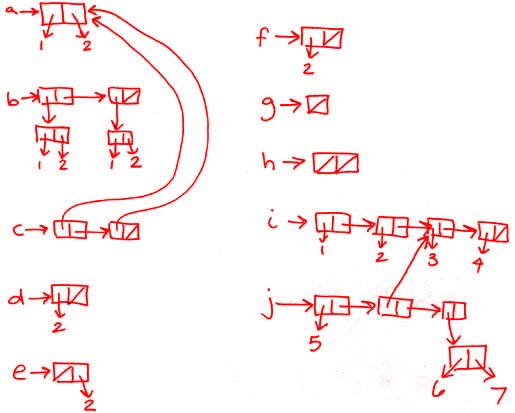```recitation 5: cons/car/cdr/list

--------------------------------------------
means of combination: compound data!
means of abstraction: data abstraction!
contract for glue & unglue
don't need to know implementation details
(black box)

cons  "constructor"
car   "contents of address portion of register"
cdr   "contects of decrement portion of register"

is cons/car/cdr/list a special form?
(car (cons (+ 3 4) (/ 4 0)))

no, you follow the normal rules of evaluation
(evaluating the expression above results in an error)

--------------------------------------------
drawing cons cell diagrams:
* any time you see cons, draw a double box with 2 pointers
* any time you see list with n items, draw a chain of n cons cells
* list with no items is nil
* evaluate the stuff inside & point to it

(define a (cons 1 2))

(define b (list (cons 1 2) (cons 1 2)))

(define c (list a a))

(define d (cons 2 nil))

(define e (cons nil 2))

(define f (list 2))

(define g (list))

(define h (list nil))

(define i (list 1 2 3 4))

(define j (list 5 (cdr (cdr i)) (cons 6 7)))* don't forget to label your diagrams with any defined variables

* arrows that point to cons cells can point to any part of the outer box,

* you can write numbers inside the boxes, or point to them outside the box

* don't forget to put something in each box (don't forget to put a
slash in the last box of a list)

--------------------------------------------
to print a cons structure:
* each cons cell is printed ( <car> . <cdr> )
* cross out any ". nil"
* cross out any ". (" and it's matching ")"
* the empty list may print out as () or #f

(cons 1 2)  =>
(1 . 2)

(cons (cons 1 2) (cons 3 4)) =>
((1 . 2) . (3 . 4))
((1 . 2) 3 . 4)

(cons 1 (cons 2 (cons 3 (cons 4 nil)))) =>
(1 . (2 . (3 . (4 . nil))))
(1 . (2 . (3 . (4))))
(1 . (2 . (3 4)))
(1 . (2 3 4))
(1 2 3 4)

(list 1 2 3 4) =>
(1 2 3 4)

The examples from above print out as follows:

a => (1 . 2)

b => ((1 . 2) . (1 . 2))
((1 . 2)  1 . 2)

c => ((1 . 2) . (1 . 2))
((1 . 2)  1 . 2)

d => (2 . nil)
(2)

e => (#f . 2)

f => (2)

g => #f

h => (#f . #f)
(#f)

i => (1 2 3 4)

j => (5 (3 4) (6 . 7))

--------------------------------------------
* whats the minimum number of cons cells needed to store n items?

n-1

--------------------------------------------
"cons-ing up a list"

(define (enumerate-interval a b)
(if (> a b) nil
(cons a
(enumerate-interval (+ 1 a) b))))

(enumerate-interval 4 9) => (4 5 6 7 8 9)

* write an iterative version:

(define (enumerate-interval a b)
(define (helper b so-far)
(if (> a b)
so-far
(helper (- b 1) (cons b so-far))))
(helper b nil))

* iterative procedures often require helper functions, but not always
* in block structure, a is known from outer procedure

--------------------------------------------
"cdr-ing down a list"

(define (length lst)
(if (null? lst)
0
(+ 1 (length (cdr lst)))))

(length (list 1 3 4 7)) => 4

* write an iterative version:

(define (length lst)
(define (helper lst count)
(if (null? lst) count
(helper (cdr lst) (+ count 1))))
(helper lst 0))

--------------------------------------------
"cdr-ing down the input & consing up a result"

Write cube-neighbor-diff:

(cube-neighbor-diff (list 1 3 4 7)) => (8 1 27)

(define (cube-neighbor-diff lst)
(cond ((null? lst) nil)
((null? (cdr lst)) nil)
(else (cons (* (- (cadr lst) (car lst))
(cube-neighbor-diff (cdr lst))))))

the special form let:
(let ((a 5)
(b 10))
(+ a b))

using let:
(define (cube-neighbor-diff lst)
(cond ((null? lst) nil)
((null? (cdr lst)) nil)
(else (let ((a (- (cadr lst) (car lst))))
(cons (* a a a)
(cube-neighbor-diff (cdr lst)))))))

```